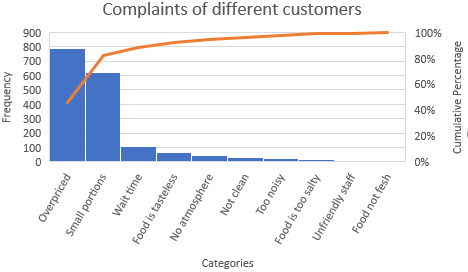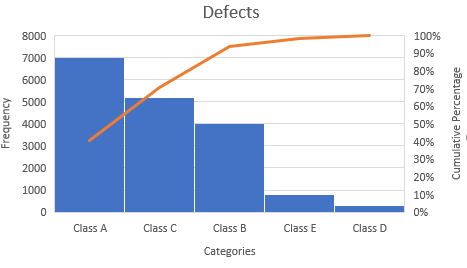# R – Pareto Chart

Pareto chart is a combination of a bar chart and a line chart used for visualization.
In Pareto charts, the right vertical axis is used for cumulative frequency while the left vertical axis represents frequency. They basically use the Pareto principle which says that 80% of effects are produced from 20% of causes of systems.

Here, we have a bar chart that indicates the frequency of occurrence of the event in different categories in decreasing order (from left to right), and an overlaid line chart indicates the cumulative percentage of occurrences.

Syntax:
pareto.chart(x, ylab = “Frequency”, ylab2 = “Cumulative Percentage”, xlab, cumperc = seq(0, 100, by = 25), ylim, main, col = heat.colors(length(x)))

Parameters:
x: a vector of values. names(x) are used for labelling the bars.
ylab: a string specifying the label for the y-axis.
ylab2: a string specifying the label for the second y-axis on the right side.
xlab: a string specifying the label for the x-axis.
cumperc: a vector of percentage values to be used as tickmarks for the second y-axis on the right side.
ylim: a numeric vector specifying the limits for the y-axis.
main: a string specifying the main title to appear on the plot.
col: a value for the color, a vector of colors, or a palette for the bars. See the help for colors and palette.

#### Plotting Pareto Chart

Following are the steps that are required for plotting Pareto Chart:

• A vector (defect <- c(Values…)) is taken which holds the values of counts of different categories.
• A vector (names(defect) <- c(Values…)) is taken which holds the string values specifying
names of different categories.
• This vector “defect” is plot using pareto.chart().

Example 1:

 `# x axis numbers ` `defect <``-` `c(``27``, ``789``, ``9``, ``65``, ``12``, ``109``, ``30``, ``15``, ``45``, ``621``) ` ` `  `# x axis titles ` `names(defect) <``-` `c(``"Too noisy"``, ``"Overpriced"``, ``"Food not fresh"``,  ` `                   ``"Food is tasteless"``, ``"Unfriendly staff"``, ` `                   ``"Wait time"``, ``"Not clean"``, ``"Food is too salty"``,  ` `                   ``"No atmosphere"``, ``"Small portions"``)  ` ` `  `pareto.chart(defect, xlab ``=` `"Categories"``, ``# x-axis label ` `                     ``ylab``=``"Frequency"``,  ``# label y left ` ` `  `# colors of the chart              ` `col``=``heat.colors(length(defect)),  ` ` `  `# ranges of the percentages at the right ` `cumperc ``=` `seq(``0``, ``100``, by ``=` `20``),   ` ` `  `# label y right ` `ylab2 ``=` `"Cumulative Percentage"``,  ` ` `  `# title of the chart ` `main ``=` `"Complaints of different customers"`  `) `

Output :In the chart here, the orange Pareto line indicates that (789 + 621) / 1722 which is approximately 80% of the complaints come from 2 out of 10 = 20% of the complaint types (Overpriced and Small portions).

Example 2:

 `# x axis numbers ` `defect <``-` `c(``7000``, ``4000``, ``5200``, ``3000``, ``800``) ` ` `  `# x axis titles ` `names(defect) <``-` `c(``"Class A"``, ``"Class B"``, ``"Class C"``, ` `                   ``"Class D"``, ``"Class E"``)  ` ` `  `pareto.chart(defect, xlab ``=` `"Categories"``, ` `                     ``ylab``=``"Frequency"``, ` `             ``col``=``heat.colors(length(defect)), ` `             ``cumperc ``=` `seq(``0``, ``100``, by ``=` `10``), ` `             ``ylab2 ``=` `"Cumulative Percentage"``, ` `             ``main ``=` `"Defects"` `) `

Output:My Personal Notes arrow_drop_upCheck out this Author's contributed articles.

If you like GeeksforGeeks and would like to contribute, you can also write an article using contribute.geeksforgeeks.org or mail your article to contribute@geeksforgeeks.org. See your article appearing on the GeeksforGeeks main page and help other Geeks.

Please Improve this article if you find anything incorrect by clicking on the "Improve Article" button below.

Article Tags :

Be the First to upvote.

Please write to us at contribute@geeksforgeeks.org to report any issue with the above content.